# Help of Geometry Problem 39. Triangle, Incircle, Cyclic QuadrilateralsSuggestions for Problem 39:

DEFINITION 1.

Midpoint is the point on a line segment dividing it into two segments of equal length.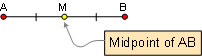DEFINITION 2. Angle is the figure formed by two rays with a common end point.

Congruent angles are angles that have the exact same measure (the same number of degrees).

Angle Bisector is a ray that divides the angle into two congruent adjacent angles.DEFINITION 3. Perpendiculars are lines or rays or segments that meet at right angles.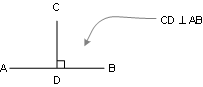DEFINITION 4. Parallel lines are straight lines which lie in the same plane and do not intersect however far they are extended.

PROPOSITION 1. If two lines are parallel, each pair of alternate interior angles are congruent. Also converse.PROPOSITION 2. If two lines are parallel, each pair of corresponding angles are congruent. Also converse.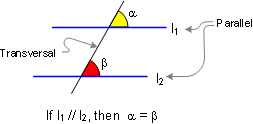DEFINITION 5. Triangle is a three side polygon. Polygon is a closed plane figure with
n sides. Altitude is the perpendicular line segment from one vertex to the line that contains the opposite side.PROPOSITION 3. The sum of the measures of the three angles of a triangle is 180.PROPOSITION 4. The measure of an exterior angle of a triangle equals the sum of the measures of the two non-adjacent interior angles.PROPOSITION 5. The sum of the measures of the acute angles of a right triangle is 90 (complementary).PROPOSITION 6. Triangle Congruence S.A.S. If two sides and the included angle of one triangle are congruent to the corresponding parts of another, then the triangles are congruent.PROPOSITION 7. Triangle Congruence A.S.A. If two angles and the included side of one triangle are congruent to the corresponding parts of another, then the triangles are congruent.PROPOSITION 8. Triangle Congruence S.S.S. If three sides of one triangle are congruent to the three sides of a second triangle, then the triangles are congruent.PROPOSITION 9. Any point on the bisector of an angle is equidistant from the sides of the angle.DEFINITION 6. Circle is the set of all points in a plane that are at the same distance from a fixed point called the center.

Tangent of a circle is a line that touches the circle at one and only one point no matter how far produced.

PROPOSITION 10. If a line is tangent to a circle, it is perpendicular to a radius at the point of tangency.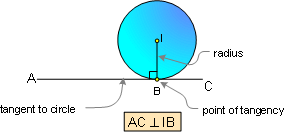PROPOSITION 11. The bisectors AD, BF and CE of the angles of a triangle ABC meet in a point I, which is equidistant from the sides of the triangle.

The incircle is the inscribed circle of a triangle. The center of the incircle is called the incenter, and the radius of the circle is called the inradius.PROPOSITION 12. Two tangent segments to a circle from an external point are congruent.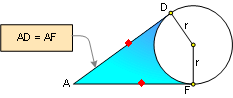PROPOSITION 13. Isosceles triangle: If two sides of a triangle are congruent, the angles opposite these sides are congruent. Also converse.PROPOSITION 14. A central angle is measured by its intercepted arc.PROPOSITION 15. An inscribed angle is measured by one-half its intercepted arc.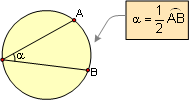PROPOSITION 16. A cyclic quadrilateral is a quadrilateral whose vertices all lie on a single circle.

16.1. Opposite angles of a cyclic (inscribed) quadrilateral are supplementary. Also converse.16.2. A quadrilateral is cyclic if one side subtends congruent angles at the two opposite vertices. Also converse.DEFINITION 7. Similar Triangles are triangles whose corresponding angles are congruent and whose corresponding sides are in proportion.

PROPOSITION 17. Triangle Similarity AA. If two angles of one triangle are congruent to two angles of another triangle, the two triangles are similar.Home | Geometry | Search | Problems | Open Problems | 10 Problems | Email | by Antonio Gutierrez